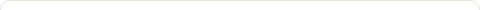# Java Program to Convert Decimal Number to Roman Number

Posted on: March 28, 2016

Objectives :

• Convert Decimal number to Roman number
• Convert Decimal numeral to Roman numeral
• How to convert a decimal number to roman number
• Write a program that converts a decimal number to Roman number.
• Write a program that converts a decimal number to Roman number. Decimal Number is accepted using Scanner class at the time of execution.
• Write a program that converts a decimal number to Roman number. Decimal Number is accepted as command line input at the time of execution.

Program :

### Java program that converts a decimal number to Roman number. Decimal Number is accepted using Scanner class at the time of execution.

```
&nbsp;

import java.util.Scanner;

public class DecimalToRoman {

private static String toRoman(int num) {
String[] romanCharacters = { "M", "CM", "D", "C", "XC", "L", "X", "IX", "V", "I" };
int[] romanValues = { 1000, 900, 500, 100, 90, 50, 10, 9, 5, 1 };
String result = "";

for (int i = 0; i < romanValues.length; i++) {
int numberInPlace = num / romanValues[i];
if (numberInPlace == 0) continue;
result += numberInPlace == 4 && i > 0? romanCharacters[i] + romanCharacters[i - 1]:
new String(new char[numberInPlace]).replace("\0",romanCharacters[i]);
num = num % romanValues[i];
}
return result;
}

public static void main(String[] args) {
Scanner scanner = new Scanner(System.in);
System.out.print("Enter a number : ");
int decimal = scanner.nextInt();
System.out.println(toRoman(decimal));
}

}

```

Output :

Enter a number : 1234

MCCXXXIV

### Java program that converts a decimal number to Roman number. Decimal Number is accepted as command line input at the time of execution.

```
public class DecimalToRoman {

private static String toRoman(int num) {
String[] romanCharacters = { "M", "CM", "D", "C", "XC", "L", "X", "IX", "V", "I" };
int[] romanValues = { 1000, 900, 500, 100, 90, 50, 10, 9, 5, 1 };
String result = "";

for (int i = 0; i < romanValues.length; i++) {
int numberInPlace = num / romanValues[i];
if (numberInPlace == 0) continue;
result += numberInPlace == 4 && i > 0? romanCharacters[i] + romanCharacters[i - 1]:
new String(new char[numberInPlace]).replace("\0",romanCharacters[i]);
num = num % romanValues[i];
}
return result;
}

public static void main(String[] args) {
if(args.length<1 || args.length>1){
System.out.println("Wrong input");
}else{
String number = args;
int decimal = Integer.parseInt(number);
System.out.println(toRoman(decimal));
}
}

}

```

### 1 Response to "Java Program to Convert Decimal Number to Roman Number"Can someone kindly explain the part below?
Really appreciate it as I’m a bit lost.

result += numberInPlace == 4 && i > 0? romanCharacters[i] + romanCharacters[i – 1]:
new String(new char[numberInPlace]).replace(“\0”,romanCharacters[i]);

Like